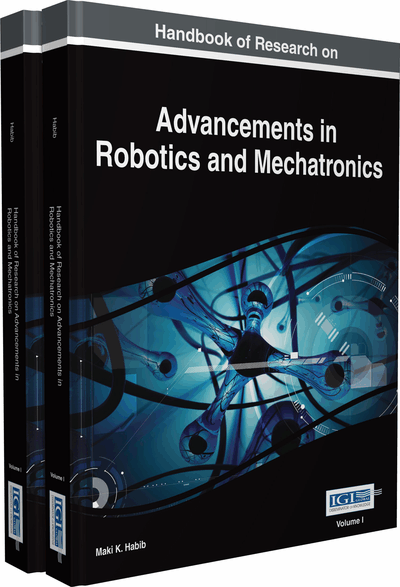# Comparison of Control Strategies by the Example of the Cooling Fan Control of a Mobile Machine

Kai Borgeest (Aschaffenburg University of Applied Sciences, Germany) and Peter Josef Schneider (Continental Teves AG & Co. oHG, Germany)
DOI: 10.4018/978-1-4666-7387-8.ch017

## Abstract

In order to compare different control strategies, the cooling system of a mobile machine has been chosen. The example control problem was to run the cooling system for m control variables and with n=m correction variables in a way to minimize power in order to save energy and to reduce fan noise while maintaining sufficient cooling. The plant is nonlinear. Three different kinds of controllers have been investigated in several variations (i.e. fuzzy control, PI[D], and Model Predictive Control [MPC]). Fourteen different criteria have been used in this chapter for evaluation. In many respects, a linear controller with fuzzy prediction proved best, in particular the prediction model can handle nonlinear properties of the plant.
Chapter Preview
Top

## Background

### PID Control

The probably oldest kind of linear controllers is a proportional controller (P controller) which generates a control action u(t) proportional to the error e(t), i.e. the deviation of the controlled variable from the reference. To eliminate the permanent remaining error e(t → ∞) of a proportional control an integrator can be switched in parallel to the proportional controller, this combination is called a PI controller. In order to accelerate the response of the controller an additional differentiator can be switched in parallel to the PI controller, this combination is called a PID controller. Let u(t) be the variable manipulated by the controller, so a PID controller is defined in time domain by

, (1) where KP, KI and KD are proportional, integral and differential coefficients and τ is the integrator time. In frequency domain the Laplace transform of (1) yields the transfer function, (2) with.

PI controllers and PID controllers are the standard tools of control engineering, because they can be easily realized as analogue electronic circuits with operational amplifiers or today as computationally efficient and amazingly simple software code on microcontrollers.

The use of digital controllers brought up several other concepts and for each application the question should be asked if another type of controller can do better. Furthermore a definition of the term “better” depending on the technical application is necessary. We will define later many criteria which define the term “better”.

## Key Terms in this Chapter

Disturbance Feedforward: The controller is informed about disturbances and compensate for them before the effect on the controlled variable shows up.

Membership Function: A function which for each fuzzy set assigns a physical value on the abscissa to a truth value which describes to which extent (between 0 and 1) this value belongs to the set.

Dumper: A construction machine to move and dump bulk solids.

Fuzzification: Assignment of an input variable to fuzzy sets.

PID Control: A strategy which uses a combination of proportional, integral and differential control.

Model Predictive Control (MPC): A controller which considers future states of variables obtained by a model.

Hardware in the Loop (HiL): Computer simulation model with true physical interfaces to the sensor/actor ports of an electronic control unit.

Defuzzification: Assignment of fuzzy values to a numerical output value.

Identification: The process to model a plant by either pure mathematical methods or a combination of physical and mathematical methods.

MIMO System: System with multiple inputs and multiple outputs.

LTI System: Linear time invariant system.

State Space: The possible space in which trajectories of internal state variables can be located. The state space evaluation can be used for a class of powerful controllers.

PWM: Pulse Width Modulation, a method to generate a floating analog average of a native digital signal by variation of the ratio between on and off time.

Fuzzy Control: A control strategy which uses discrete value ranges of a variable which can be characterized by linguistic terms, such as “hot” or “cold”.

## Complete Chapter List

Search this Book:
Reset